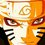# Olympiad Proof Problem - Day 6

It had been long days I had posted Olympiad Proof Problem - Day 5. Sorry for the inconvenience made to those who are waiting for the Proof Problem Day - 6. So, Finally here is the proof problem day - 6.

If $x_{1}$, $x_{2}$, $x_{3}$, $\ldots$ $x_{n}$ are positive real numbers, then prove the following inequality

$\Large \sum_{i=1}^{n} \dfrac{x_{i} ^3}{x_{i}^2 + x_{i}x_{i+1} + x_{i+1}^2} \geq \dfrac{x_{1} + x_{2} + x_{3} + \ldots + x_{n}}{3}$

Details and Assumptions:

• $x_{n+1} = x_{1}$.

###### Try more proof problems at Olympiad Proof Problems.Note by Surya Prakash
5 years, 8 months ago

This discussion board is a place to discuss our Daily Challenges and the math and science related to those challenges. Explanations are more than just a solution — they should explain the steps and thinking strategies that you used to obtain the solution. Comments should further the discussion of math and science.

When posting on Brilliant:

• Use the emojis to react to an explanation, whether you're congratulating a job well done , or just really confused .
• Ask specific questions about the challenge or the steps in somebody's explanation. Well-posed questions can add a lot to the discussion, but posting "I don't understand!" doesn't help anyone.
• Try to contribute something new to the discussion, whether it is an extension, generalization or other idea related to the challenge.

MarkdownAppears as
*italics* or _italics_ italics
**bold** or __bold__ bold
- bulleted- list
• bulleted
• list
1. numbered2. list
1. numbered
2. list
Note: you must add a full line of space before and after lists for them to show up correctly
paragraph 1paragraph 2

paragraph 1

paragraph 2

[example link](https://brilliant.org)example link
> This is a quote
This is a quote
    # I indented these lines
# 4 spaces, and now they show
# up as a code block.

print "hello world"
# I indented these lines
# 4 spaces, and now they show
# up as a code block.

print "hello world"
MathAppears as
Remember to wrap math in $$ ... $$ or $ ... $ to ensure proper formatting.
2 \times 3 $2 \times 3$
2^{34} $2^{34}$
a_{i-1} $a_{i-1}$
\frac{2}{3} $\frac{2}{3}$
\sqrt{2} $\sqrt{2}$
\sum_{i=1}^3 $\sum_{i=1}^3$
\sin \theta $\sin \theta$
\boxed{123} $\boxed{123}$

Sort by:

Observe that

$0 = (x_1 - x_2) + (x_2 - x_3) + \ldots + (x_n - x_1) = \displaystyle \sum_{i=1}^n \dfrac {x_{i}^3 - x_{i+1}^3}{x_i^2+x_i x_{i+1}+x_{i+1}^2}$

Hence,

$\displaystyle \sum_{i=1}^n \dfrac {x_i^3}{x_i^2+x_ix_{i+1}+x_{i+1}^2} = \dfrac {1}{2} \displaystyle \sum_{i=1}^n \dfrac {x_i^3+x_{i+1}^3}{x_i^2+x_ix_{i+1}+x_{i+1}^2}$

Note that $a^3 + b^3 \geq \frac{1}{3} a^3 + \frac{2}{3} a^2b + \frac {2}{3} ab^2 + \frac{1}{3} b^3 = \frac {1}{3} (a+b)(a^2+ab+b^2)$ (which can be easily proven by AM-GM). From this, we attain:

$\dfrac {1}{2} \displaystyle \sum_{i=1}^n \dfrac {x_i^3+x_{i+1}^3}{x_i^2+x_ix_{i+1}+x_{i+1}^2} \geq \dfrac {1}{2} \displaystyle \sum_{i=1}^n \dfrac {x_1+x_{i+1}}{3}=\dfrac {1}{3} \displaystyle \sum_{i=1}^n x_i$

which is the answer we wished to obtain.

- 5 years, 8 months ago

Cool!! Nice solution. But you should have waited some more time to post the solution. So that this note would have reached to many people.

- 5 years, 8 months ago

Sorry.

- 5 years, 8 months ago

It's ok.

- 5 years, 8 months ago

For those who want the AM-GM proof, here it is (I'm working backwards but you can see what you must do to get the identity):

$a^2 + b^2 \geq 2ab$

$a^2+b^2 - ab \geq ab$

$(a+b)(a^2+b^2-ab) \geq ab(a+b)$

$a^3 + b^3 \geq a^2 b + ab^2$

$\dfrac {2}{3} a^3 + \dfrac {2}{3} b^3 \geq \dfrac {2}{3} a^2 b + \dfrac {2}{3} ab^2$

$a^3 + b^3 \geq \dfrac {1}{3} a^3 + \dfrac {1}{3} b^3 + \dfrac {2}{3} a^2 b + \dfrac {2}{3} ab^2$

which is the identity. Thus proven.

- 5 years, 8 months ago

Good Good

- 5 years, 8 months ago